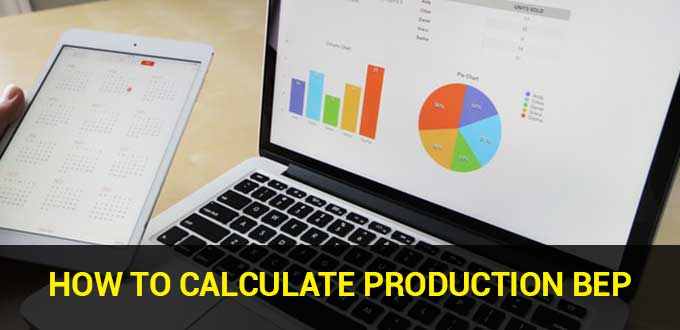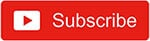# What is Production BEP and How is Production BEP Calculated?Production BEP is the point at which the varied total expenditures incurred for the production process balance with the total income at the end of the financial recording period, without the company receiving net profit or loss.

The most crucial thing to remember while doing business is to start calculating BEP. So, exactly what is a break even point? The break even point is a mathematical point at which the quantity of capital supplied equals the amount of income. In other words, the corporation makes no money.

The break even point is one of the aspects that must be addressed while running a business so that you know when the business will double its profits as long as it is operating.

## The BEP FormulaIn practice, various factors influence the calculation of the BEP formula, namely:

### 1. Cost components that have been entered as fixed costs

BEP is calculated by dividing fixed costs by the price per unit of product minus variable expenses.

Meanwhile, the sales BEP in Rupiahs is determined using the formula for fixed costs divided by 1 minus variable costs after dividing by sales volume.

As an entrepreneur, you need to know what components can be included in fixed expenses based on the calculation above. Fixed costs are nominal amounts that are constant and do not vary with the volume of output carried out.

Fixed costs include rent for a manufacturing operational building, personnel pay, energy, internet, telephone, and water fees, as well as initial raw material prices. It’s merely that when production volume expands to satisfy market demand, the cost of raw materials, electricity, or the internet can become changeable.

### 2. What are the different types of variable costs?

One of the advantages of BEP is recognizing what types of items are in high demand in the market and expanding production of such things.

Furthermore, when the BEP formula is appropriately implemented, it allows the firm’s management to innovate on other products that are also manufactured by the company but are still low in consumer demand.

Regardless of the type of product produced, this is also related to variable costs, where variable expenses should not cover more than 50% of the company’s operational expenditures in order to reach BEP promptly.

Variable costs are often tied to the nominal amount, which always varies with market demand for commodities.

### 3. Profit percentage to be attained

Production BEP is one of the components that must be grasped in theory before establishing the selling price. However, the projected percentage of profit you intend to make when selling a specific product is critical since it influences how quickly the company can accomplish BEP.

If you don’t want to lose money, you can use a simple application like the iReap cashier or a similar application to learn how much the selling price should be set at the beginning of the business for branding, while also attracting the attention of consumers who are still unfamiliar with your product.

### 4. The portion of the company’s sales and marketing financing

BEP and sales marketing are inextricably linked. Production BEP is a component whose calculation is proportionate to the sales statistics required for product branding.

## Analysis of the Break Even PointTo understand how to compute production BEP, you must first understand the fundamental concepts of break even analysis. What exactly is the purpose of BEP? The BEP function is important for determining the amount of sales volume that will later seek to earn profits or losses.

The primary advantage of calculating BEP is that it is effective for generating a margin that will subsequently function as a barrier to prevent losses.

Furthermore, BEP plays a crucial function at the start of the investment, so you know how much investment you need to have so that you can balance the amount of capital costs utilized as initial expenses later on.

BEP also plays a significant part in the purchase and sale of shares, namely the analysis of various projects carried out by a corporation.## How to Determine Production BEP

If you study accounting, you will utilize BEP as an equation to compute the cost of spending on items based on the costs you will receive. The BEP calculating procedure is an important instrument for issue solving in the accounting profession.

So, the formula for computing BEP is as follows: Fixed costs = (sale price of product units – variable unit products).

There is a difference in the formula between the unit selling price and the product variable. This is a formula for calculating the contribution margin. This method can be used if you wish to determine the point at which your total expenses equal your total costs and the number of units issued in your business.

BEP for Dual Products = Fixed cost/[(1-v/c) x Wi] is the break even point utilized in dual products. The V/C ratio in the Double BEP calculation compares variable costs to the product’s selling price.

The unit contribution method is another approach for calculating BEP. The total contribution margin, which is the difference between revenue, product sales, and variable costs, is used to determine this technique.

Using the unit contribution approach, you can generally calculate how much profit you will make from the things you sell. BEP stands for fixed cost/contribution margin.

You can use the graphical technique in addition to the methods already stated, but there is an easier option that will not disturb you, notably by using iReap, an application that can be utilized during the checkout process of a sale.